How Cheenta works to ensure student success?
Explore the Back-Story

# Test of Mathematics Solution Subjective 166 -The Grazing FieldThis is a Test of Mathematics Solution Subjective 166 (from ISI Entrance). The book, Test of Mathematics at 10+2 Level is Published by East West Press. This problem book is indispensable for the preparation of I.S.I. B.Stat and B.Math Entrance.

Also visit: I.S.I. & C.M.I. Entrance Course of Cheenta

## Problem

A cow is grazing with a rope around its neck and the other end of the rope is tied to a pol. The length of the rope is 10 metres. There are two boundary walls perpendicular to each other, one at a distance of 5 metres to the east of the pole and another at a distance of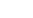metres to the north of the pole. Find the area the cow can graze on.

## Solution

First we need to analyse the question and draw a diagram. The figure for the problem would look something like thisWe have a circle with centre P and radius 10m. Let AC and AB be the boundary walls present. The striped region shows the area available for grazing. Now if we see carefully we can see that area of the shaded region is nothing but the sum of the areas of the rectangle AEPC, the two right angled triangles EPB and DPC and the sector BPC.

PB = PC = radius of the circle = 10m

PE =m

AS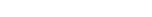(Pythagorean property of a right angled triangle)

From here, we get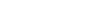m

Similarly, as PD = 5m, PC = 10mm              (pythagorean property)

Therefore,

Area of rectangle AEPC =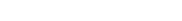Area of triangle PEB =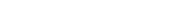Area of triangle PDC =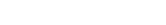Area of sector BPC =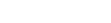Now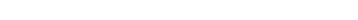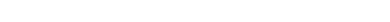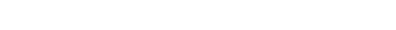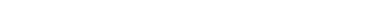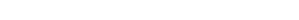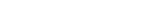Therefore area of sector BPC =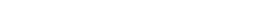Thus Total area of the Grazing Field =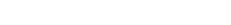or to simplify Total Area =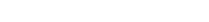This is a Test of Mathematics Solution Subjective 166 (from ISI Entrance). The book, Test of Mathematics at 10+2 Level is Published by East West Press. This problem book is indispensable for the preparation of I.S.I. B.Stat and B.Math Entrance.

Also visit: I.S.I. & C.M.I. Entrance Course of Cheenta

## Problem

A cow is grazing with a rope around its neck and the other end of the rope is tied to a pol. The length of the rope is 10 metres. There are two boundary walls perpendicular to each other, one at a distance of 5 metres to the east of the pole and another at a distance ofmetres to the north of the pole. Find the area the cow can graze on.

## Solution

First we need to analyse the question and draw a diagram. The figure for the problem would look something like thisWe have a circle with centre P and radius 10m. Let AC and AB be the boundary walls present. The striped region shows the area available for grazing. Now if we see carefully we can see that area of the shaded region is nothing but the sum of the areas of the rectangle AEPC, the two right angled triangles EPB and DPC and the sector BPC.

PB = PC = radius of the circle = 10m

PE =m

AS(Pythagorean property of a right angled triangle)

From here, we getm

Similarly, as PD = 5m, PC = 10mm              (pythagorean property)

Therefore,

Area of rectangle AEPC =Area of triangle PEB =Area of triangle PDC =Area of sector BPC =NowTherefore area of sector BPC =Thus Total area of the Grazing Field =or to simplify Total Area =### Knowledge Partner